# 5.3 Number, String, or Boolean? Datatype Conversion

As defined earlier, JavaScript is a loosely typed language, which really means that you don't have to be concerned about what kind of data is stored in a variable. You can assign a number to x on one line and on the next line assign a string to x , you can compare numbers and strings, strings and Booleans, and so on. JavaScript automatically converts values when it assigns values to a variable or evaluates an expression. If data types are mixed (i.e., a number is compared with a string, a Boolean is compared with a number, a string is compared with a Boolean), JavaScript must decide how to handle the expression. Most of the time, letting JavaScript handle the data works fine, but there are times when you want to force a conversion of one type to another. For example, if you prompt a user for input, the input is set as a string. But, suppose you want to perform calculations on the incoming data, making it necessary to convert the strings to numbers . When using the + operator you want to add two numbers that have been entered as strings, not concatenate them, so you will then need to convert the data from string to number.

JavaScript provides three methods to convert the primitive data types. They are:

• String()

• Number()

• Boolean()

##### Example 5.14
` <html>     <head><title>The Conversion Methods</title></head>     <body>     <p>     <h3>Data Conversion</h3>     script language="JavaScript"> 1       var num1 = prompt("Enter a number: ","");         var num2 = prompt("Enter another number: ",""); 2  var result = Number(num1) + Number(num2);  //  Convert strings to numbers  3       alert("Result is "+  result  ); 4  var myString=String(num1);  5  result=myString + 200;  //  String + Number is String  6       alert("Result is "+ result);   //  Concatenates 200 to the  //  result; displays 20200  7  alert("Boolean result is "+ Boolean(num2));  //  Prints true  </script>     </body>     </html> `

EXPLANATION

1. The user is prompted to enter a number (see Figure 5.20). The variable num1 is assigned the number. On the next line, num2 is assigned another number entered by the user (see Figure 5.21).

##### Figure 5.20. The user is prompted to enter a number.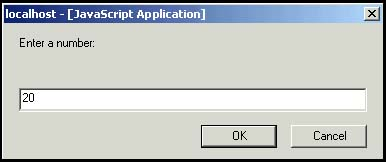##### Figure 5.21. The user is prompted to enter another number.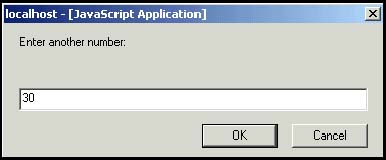2. The JavaScript Number() method converts strings to numbers. After the variables , num1 and num2 have been converted to numbers, the + sign will be used as an addition operator (rather than a concatenation operator), resulting in the sum of num1 and num2 . Unless converted to numbers, the string values 30 + 20 would be concatenated , resulting in 3020 .

3. The alert() box displays the sum of the two numbers entered by the user (see Figure 5.22).

##### Figure 5.22. The result is displayed.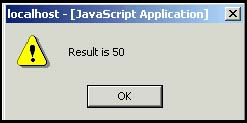4. The variable num1 is converted to a string; its value is assigned to the variable, result .

5. The value of myString, 20, is concatenated to 200 and assigned to result . The result is 20200 .

6. The alert() box displays the result from line 5.

7. The value of num2 is converted to Boolean, either true or false . Since the value of num2 is not 0, true is displayed in the alert() dialog box.

#### 5.3.1 The parseInt() Method

This method converts a string to a number. It starts parsing at the beginning of the string and returns all integers until it reaches a non-integer and then stops parsing. If the string doesn't begin with an integer, NaN  (not a number) is returned. For example, parseInt("150cats") becomes 150 , whereas parseInt("cats") becomes NaN . You can also use octal and hexadecimal numbers. In the two-argument format, the first argument to parseInt() is a string containing a number base (radix) ranging from 2 to 36. The default is base 10. In the statement, parseInt("17", 8) , the result is 15 . The first argument is the string to be parsed and the second argument, 8, is the number base of the number (here, octal 17). The value returned is decimal 15 . Refer to Tables 5.14 and 5.15.

 NaN is not supported in JavaScript 1.0; instead of NaN , 0 is returned.

FORMAT

` `
` parseInt(String, NumberBase);  Default base is 10  parseInt(String); `

Example:

` `
` parseInt("111", 2);    7  (  111 in base 2 is 7)  parseInt("45days");  45  `

String

Result

"hello"

NaN

"Route 66"

NaN

"6 dogs"

6

"6"

6

"-6"

“6

"6.56"

6

"0Xa"

10

"011"

9

String

Base

Result (decimal)

"111"

2 (binary)

7

"12"

8 (octal)

10

"b"

16 (hex)

11

##### Example 5.15
` <html>     <head>     <title>Using the parseInt() Function</title></head>     <body><font face="arial size="+1">     <b>     <script language = "JavaScript"> 1       var grade = prompt("What is your grade? ", "");                // Grade entered as a string 2  grade=parseInt(grade);  // Grade converted to an integer 3       document.write("grade type is<em> " +  typeof(grade))  ; 4       grade+=10; 5       document.write("<em><br>After a 10 point bonus, your grade is "                        + grade + "!<br>");     </script>     </body>     </html> `

EXPLANATION

1. The user is prompted to enter a grade. The string value entered in the prompt box is assigned to the variable grade . (See Figure 5.23.)

##### Figure 5.23. The user enters a grade.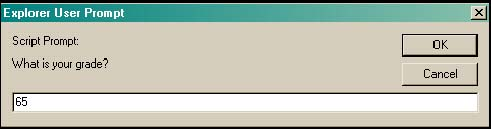2. The parseInt() method will convert the grade to an integer value.

3. The typeof() operator returns the data type of the variable grade .

4. The value of grade is incremented by 10.

5. The new value of grade is sent to the browser. (See Figure 5.24.)

##### Figure 5.24. The new value of grade is displayed in the browser.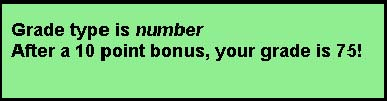#### 5.3.2 The parseFloat() Method

The parseFloat() method is just like the parseInt() method except that it returns a floating-point number. A floating-point  number is a number that contains a fractional part, such as 3.0, “22.5, or .15. The decimal point is allowed in the string being parsed. If the string being parsed does not start with a number, NaN (not a number) is returned.

 The term "floating point" means that there are not a fixed number of digits before or after the decimal point; the decimal point floats.

FORMAT

` `
` parseFloat(String); `

Example:

` `
` parseFloat("45.3 degrees"); `

String

Result

"hello"

NaN

"Route 66.6"

NaN

"6.5 dogs"

6.5

"6"

6

"6.56"

6.56

##### Example 5.16
` <html>     <head>     <title>Using the parseFloat() Function</title>     <script language = "JavaScript"> 1          var temp = prompt("What is your temperature? ", ""); 2  temp=parseFloat(temp);  3          if(temp == 98.6){ 4             alert("Your temp is normal");            } 5          else{               alert("You are sick!");            }     </script></head><body></body></html> `

EXPLANATION

1. The user is prompted for input and the result is assigned as a string to the variable temp . (See Figure 5.25.)

##### Figure 5.25. User enters a string. The parseFloat() method will convert it to a floating-point number.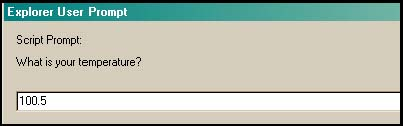2. The parseFloat() method converts the string into a floating-point number and assigns it to temp .

3. Although we haven't formally covered if statements, this example should be easy to follow. If the value of temp is equal to 98.6 , then the following block of statements will be executed.

4. If is the user entered 98.6, the alert box sends the message " Your temp is normal " to the browser.

5. If line 3 is not true, the block of statements following else is executed. An alert box will appear in the browser window saying, " You are sick! ". (See Figure 5.26.)

##### Figure 5.26. Output from Example 5.16.#### 5.3.3 The eval() Method

The eval() method evaluates a string of JavaScript statements and evaluates the whole thing as a little program, returning the result of the execution.  If there is no result, undefined is returned.

 The eval() method takes a primitive string as its argument, not a String object. If a String object is used, it will be returned as is.

FORMAT

` `
` eval(String); `

Example:

` `
` eval("(5+4) / 3"); `
##### Example 5.17
` <html>     <head>     <title>The eval() Function</title>     </head>     <body bgcolor="lightblue">     <font size="+1" face="arial">     <script language="JavaScript"> 1       var str="5 + 4"; 2  var num1 = eval(str);  3  var num2 = eval(prompt("Give me a number ", ""));  4  alert(num1 + num2);  </script>     </body>     </html> `

EXPLANATION

1. The string "5 + 4" is assigned to the variable str .

2. The eval() method evaluates the string expression "5 + 4" as a JavaScript instruction. The variable num1 is assigned 9 , the sum of 5 + 4 .

3. The eval() method evaluates the string value that is entered into the prompt dialog box. (See Figure 5.27.) The prompt() method always returns a string value. If the value in the string is a number, eval() will convert the string to a number, return the number, and assign it to num2 .

##### Figure 5.27. The eval() method converts the user input, a string, to a number.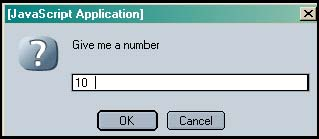4. The alert() method displays the sum of num1 and num2 in the browser window. (See Figure 5.28.)

##### Figure 5.28. Output from Example 5.17.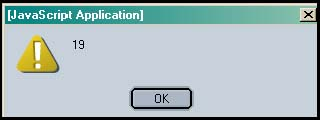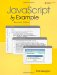JavaScript by Example (2nd Edition)
ISBN: 0137054890
EAN: 2147483647
Year: 2003
Pages: 150
Authors: Ellie Quigley

Similar book on Amazon